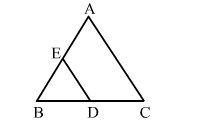# ∆ABC and ∆BDE are two equilateral triangles such that D is the midpoint of BC.

Question:

ABC and ∆BDE are two equilateral triangles such that D is the midpoint of BC. Then, ar(∆BDE) : ar(∆ABC) = ?

(a) $1: 2$

(b) $1: 4$

(c) $\sqrt{3}: 2$

(d) $3: 4$Solution:

(b) 1:4

ABC and ∆BDE are two equilateral triangles​ and D is the midpoint of BC.
Let AB = BC = AC =  a

Then $\mathrm{BD}=B E=E D=\frac{a}{2}$

$\therefore \frac{\operatorname{ar}(\Delta B D E)}{\operatorname{ar}(\Delta A B C)}=\frac{\frac{\sqrt{3}}{4} A B^{2}}{\frac{\sqrt{3}}{4} B E^{2}}=\frac{\left(\frac{a}{2}\right)^{2}}{a^{2}}=\frac{1}{4}$

So, required ratio $=1: 4$